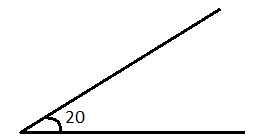QUESTION

# Find the complement of the angles represented in the given figure.Hint:-Before solving this question, we must be familiar with the term Complementary Angles.
COMPLEMENTARY ANGLES: Complementary Angles are the angles that sum up to make an angle of 90°, i.e. a right angle.Here, in this question, we need to find the complement of an angle of 20°. That means that we need to find an angle that, when added to 20°, gives an angle of 90°.